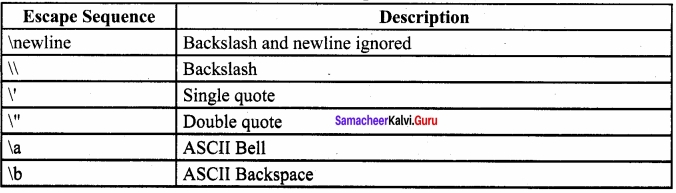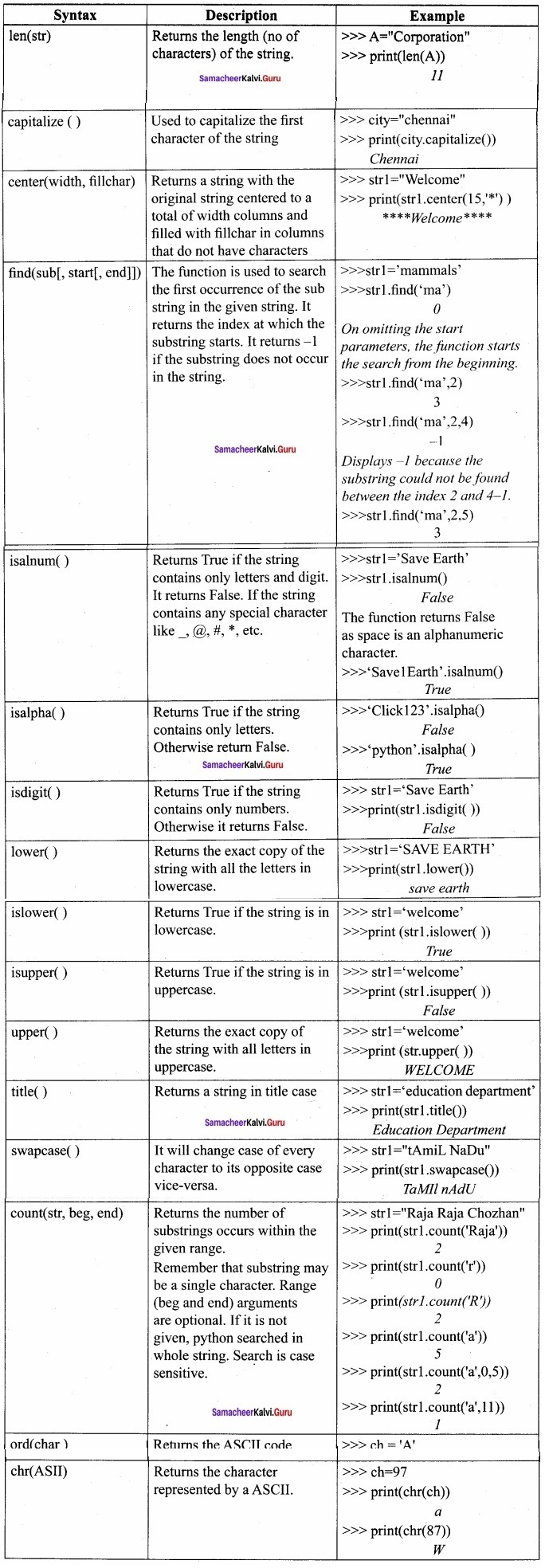Students can Download Computer Science Chapter 8 Strings and String Manipulations Questions and Answers, Notes Pdf, Samacheer Kalvi 12th Computer Science Book Solutions Guide Pdf helps you to revise the complete Tamilnadu State Board New Syllabus and score more marks in your examinations.

## Tamilnadu Samacheer Kalvi 12th Computer Science Solutions Chapter 8 Strings and String Manipulations

### Samacheer Kalvi 12th Computer Science Strings and String Manipulations Text Book Back Questions and Answers

PART – I

Question 1.
Which of the following is the output of the following python code?
print (str1 [:: -1])
(b) Tmlau
(c) UdanlimaT
(d) UdaNlimaT
(c) UdanlimaT

Question 2.
What will be the output of the following code?
str1= “Chennai Schools”
str1 = “_”
(a) Chennai – Schools
(b) Chenna – School
(c) Type error
(d) Chennai
(c) Type errorQuestion 3.
Which of the following operator is used for concatenation?
(a) +
(b) &
(c) *
(d) =
(a) +

Question 4.
Defining strings within triple quotes allows creating:
(a) Single line Strings
(b) Multiline Strings
(c) Double line Strings
(d) Multiple Strings
(b) Multiline Strings

Question 5.
Strings in python:
(a) Changeable
(b) Mutable
(c) Immutable
(d) Flexible
(c) ImmutableQuestion 6.
Which of the following is the slicing operator?
(a) { }
(b) [ ]
(c) <>
(d) ( )
(b) [ ]

Question 7.
What is stride?
(a) Index value of slide operation
(b) First argument of slice operation
(c) Second argument of slice operation
(d) Third argument of slice operation
(d) Third argument of slice operationQuestion 8.
Which of the following formatting character is used to print exponential notation in upper case?
(a) % e
(b) % E
(c) % g
(d) % n
(b) % E

Question 9.
Which of the following is used as placeholders or replacement fields which get replaced along with format ( ) function?
(a) { }
(b) <>
(c) ++
(d) ^^
(a) { }Question 10.
The subscript of a string may be:
(a) Positive
(b) Negative
(c) Both (a) and (b)
(d) Either (a) or (b)
(d) Either (a) or (b)

PART – II

Question 1.
What is String?
String is a data type in python, which is used to handle array of characters. String is a sequence of Unicode characters that may be a combination of letters, numbers, or special symbols enclosed within single, double or even triple quotes.
Example:
‘Welcome to learning Python’
“Welcome to learning Python”
“Welcome to learning Python”Question 2.
Do you modify a string in Python?
If you want to modify the string, a new string value can be assign to the existing string variable. To define a new string value to the existing string variable. Python completely overwrite new string on the existing string.
Example:
>>> str1=”How are you”
>>> print (str1)
How are you
>>> print (str1)Question 3.
How will you delete a string in Python?
Python will not allow deleting a particular character in a string. Whereas you can remove entire string variable using del command.
Example: Code lines to delete a string variable
>>> print (str1)
>>> del str1
>>> print (str1)
NameError: name ‘str1’ is not defined

Question 4.
What will be the output of the following python code?
str1 = “School”
print (str1*3)
Output:
School School SchoolQuestion 5.
What is slicing?
String slicing:
Slice is a substring of a main string. A substring can be taken from the original string by using [ ] operator and index or subscript values. Thus, [ ] is also known as slicing operator. Using slice operator, we can slice one or more substrings from a main string.

General format of slice operation:
str[start:end]
Where start is the beginning index and end is the last index value of a character in the string. Python takes the end value less than one from the actual index specified. For example, if you want to slice first 4 characters from a string, you have to specify it as 0 to 5. Because, python consider only the end value as n – 1.
Example: slice a single character from a string
>>> str1=”THIRUKKURAL”
>>> print (str1)
T

PART – III

Question 1.
Write a Python program to display the given pattern?
C O M P U T E R
C O M P U T E
C O M P U T
C O M P U
C O M P
C O M
C O
C
Program:
str1 = “COMPUTER”
index = len (str1)
for i in str 1:
print (str 1[: index])
index – = 1Question 2.
Write a short about the followings with suitable example?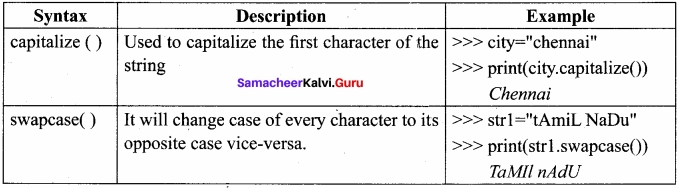Question 3.
What will be the output of the given python program?
str1 = “welcome”
str2 = “to school”
str3 = str1[: 2] str2[len(str2)-2:]
print (str3)
output:
weoo 1Question 4.
What is the use of format( )? Give an example?
The format( ) function used with strings is very versatile and powerful function used for formatting strings. The curly braces { } are used as placeholders or replacement fields which get replaced along with format( ) function.
Example:
num1 = int (input (“Number 1: “))
num2 = int (input (“Number 2: “))
print (“The sum of { } and { } is { }”.format (num1, num2,(num1 + num2)))
OutPut:
Number 1 : 34
Number 2 : 54
The sum of 34 and 54 is 88.

Question 5.
Write a note about count ( ) function in python?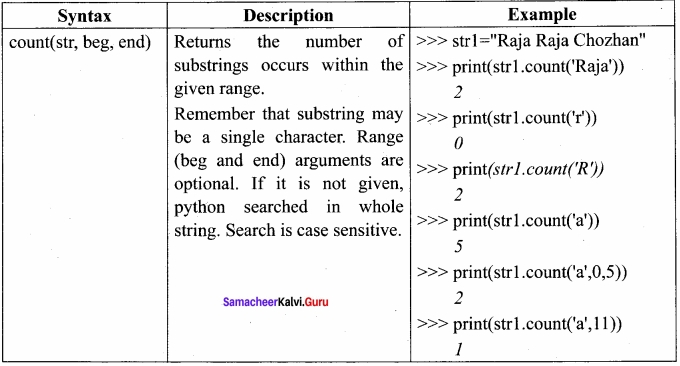PART – IV

Question 1.
Explain about string operators in python with suitable example?
String Operators:
Python provides the following operators for string operations. These operators are useful to manipulate string.

(i) Concatenation (+):
Joining of two or more strings is called as Concatenation. The plus (+) operator is used to concatenate strings in python.
Example:
>>> “welcome” + “Python”
‘welcomePython’

(ii) Append (+=):
Adding more strings at the end of an existing string is known as append. The operator += is used to append a new string with an existing string.
Example:
>>> str1 =”Welcome to ”
>>> str1+=”Leam Python”
>>> print (str1)
Welcome to Learn Python

(iii) Repeating (*):
The multiplication operator (*) is used to display a string in multiple number of times.
Example:
>>> str1 =”Welcome”
>>> print (str1*4)
Welcome Welcome Welcome Welcome

(iv) String slicing:
Slice is a substring of a main string. A substring can be taken from the original string by using [ ] operator and index or subscript values. Thus, [ ] is also known as slicing operator. Using slice operator, you have to slice one or more substrings from a main string.
General format of slice operation:
str[start: end]
Where start is the beginning index and end is the last index value of a character in the string. Python takes the end value less than one from the actual index specified. For example, if you want to slice first 4 characters from a string, you have to specify it as 0 to 5. Because, python consider only the end value as n – 1.
Example:
(i) slice a single character from a string
>>> str1=”THIRUKKURAL ”
>>> print (str1 )
T .

(v) Stride when slicing string
When the slicing operation, you can specify a third argument as the stride, which refers to the number of characters to move forward after the first character is retrieved from the string. The default value of stride is 1.
Example:
>>> str1= “Welcome to learn Python”
>>> print (str1 [10:16])
learn
Note: Remember that, python takes the last value as n – 1
You can also use negative value as stride (third argument). If you specify a negative value, it prints in reverse order.
Example:
>>> str1 = “Welcome to learn Python”
>>> print(str1 [::-2])
nhy re teoIW

Practice Programs

Question 1.
Write a python program to find the length of a string?
str=input (“Enter a string: “)
print (len(str))
Output:
Enter a string: HELLO
5Question 2.
Write a program to count the occurrences of each word in a given string?
def word_count(str):
counts = dict ( )
words = str.split ( ) for word in words:
if word in counts:
counts[word] +=1
else:
counts[word]=1
return counts
print (word_count (‘the quick brown fox jumps over the lazy dog.’))
Ouput:
{‘the’: 2, ‘jumps’: 1, ‘brown’: 1, ‘lazy’: 1, ‘fox’: 1, ‘over’: 1, ‘quick’: 1, ‘dog’: 1}Question 3.
Write a program to add a prefix text to all the lines in a string?
import
text =
“‘Strings are immutable. Slice is a
substring of a main string. Stride
is a third argument in slicing operation'”
text_without_lndentation= textwrap.dedent (text)
wrapped = extwrap.fill (text_without_Indentation, width = 50)
print (textwrap.indent(wrapped, ‘*’)
print ()
Output:

• Strings are immutable. Slice is a
• substring of a main string. Stride
• is a third argument in slicing operation

Question 4.
Write a program to print integers with ‘*’ on the right of specified width?
x = 1 2 3
print (“original number: “, x)
print (“formatted number(right padding, width 6): “+” {: * < 7 d}”.format(x));
Output:
original number : 1 2 3
formatted number (right padding, width 6): 1 2 3 ***Question 5.
Write a program to create a mirror of the given string. For example, “wel” = “lew“?
str1 = input (“Enter a string: “)
str2 = ‘ ‘
index= -1
for i in str1:
str2 += str1 [index]
index -= 1
print (“The given string = { } \n The Reversed string = { }”.format(str 1, str 2))
Output:
Enter a string: welcome
The given string = welcome
The Reversed string = emoclewQuestion 6.
Write a program to removes all the occurrences of a given character in a string?
def removechar(s,c):
# find total no of occurrence of a character
counts = s.count(c)
# convert into list of characters
s = list(s)
# keep looping until counts become 0
while counts:
# remove char, from list
s.remove(c)
counts -= 1
# join remaining characters s = ” .join(s)
print(s)
s = “python programming”‘
remove char(s, ‘p’)
Output:
ython rogramming

Question 7.
Write a program to append a string to another string without using + = operator?
s1 = input (“Enter the first string: “)
s2 = input (“Enter the second string: “)
print (‘concatenated strings =’,” ” ,join ([s1, s2]))
Output:
Enter the first string: TamilQuestion 8.
Write a program to swap two strings?
print (“Enter Y for exit.”)
string1 = input(“Enter first string: “)
if string1 = = ‘x’:
exit();
else:
string2 = input (“Enter second string : “)
print (” \n Both strings before swap : “)
print (“First string = “, string1)
print (” Second string = “, string2)
temp = string1
string1 = string2
string2 = temp
print (“\n Both strings after swap: “)
print (“First string = “, string1)
print (” Second string = “, string2)
Output:
Enter ‘x’ for exit
Enter first string: code
Enter second string: python
Both strings before swap:
First string = code
Second string = python
Both strings after swap:
First string = python
Second string = codeQuestion 9.
Write a program to replace a string with another string without using replace ( )?
s1 = input (“Enter the string to be replaced: “)
s2 = input (“Enter the string to replace with “)
s1 = s2
print (“Replaced string is “, s1)
Output:
Enter the string to be replaced: Computer
Enter the string to replace with: repcomputer
Replaced string is repcomputerQuestion 10.
Write a program to count the number of characters, words and lines in a given string?
string = input (“Enter string:”)
char = 0
word = 0
line = 0
for i in string:
char = char + 1
if (i = = “):
word = word + 1
elif (i = = ‘ \n’):
line = line +1
print (“Number of words in the string: “)
print (word)
print (“Number of characters in the string: “)
print (char)
print (“Number of lines in the string: “)
print (line)
Output:
Enter string: welcome to learning python
Number of words in the string : 4
Number of characters in the string : 26
Number of lines in the string : 1

### Samacheer kalvi 12th Computer Science Strings and String Manipulations Additional Questions and Answers

PART – 1

Question 1.
Strings in python can be created using ………………………….. quotes
(a) Single
(b) Double
(c) Triple
(d) All the above
(d) All the above

Question 2.
Strings which contains double quotes should be defined with …………………….. quotes
(a) Single
(b) Double
(c) Triple
(d) All the these
(c) TripleQuestion 3.
The positive subscript of the string starts from ………………………….. and ends with …………………………
0, n – 1

Question 4.
In strings, the negative index assigned from the last character to the first character in reverse order begins with …………………………
(a) 0
(b) 1
(c) -1
(d) -2
(c) -1

Question 5.
How will you modify the string?
(a) A new string value can be assigned to the existing string variable
(b) Updating the string character by character
(a) A new string value can be assigned to the existing string variableQuestion 6.
Which function is used to change all occurrences of a particular character in a string?
(a) Replace ( )
(b) Change ( )
(c) Edit ( )
(d) Append ( )
(a) Replace ( )

Question 7.
Which command is used to remove the entire string variable?
(a) Remove
(b) Del
(c) Delete
(d) Strike
(b) Del

Question 8.
Joining of two or more strings is called as …………………………..
(a) Append
(b) Repeating
(c) Concatenation
(d) Strike
(c) ConcatenationQuestion 9.
Adding more strings at the end of an existing strings is ………………………….
(a) Append
(b) Concatenation
(c) Repeating
(d) Slicing
(a) Append

Question 10.
Find the wrongly matched pair from the following.
(a) Append ⇒ + =
(b) Concate ⇒ +
(c) Repeat ⇒ /
(d) Slice ⇒ [ ]
(c) Repeat ⇒ /

Question 11.
Which operator is used to append a new string with an existing string?
(a) +
(b) + =
(c) *
(d) * =
(b) + =Question 12.
The multiplication operator is also called as ………………………….
(a) Append
(b) Concatenate
(c) Repeat
(d) Slice
(c) Repeat

Question 13.
Which is used to display a string multiple number of times?
(a) Repeating
(b) *
(c) Multiplication operator
(d) All the above
(d) All the above

Question 14.
…………………………. is a substring of a main string.
SliceQuestion 15.
In python, end value is considered as ……………………….
(a) 0
(b) n
(c) n – 1
(d) 1
(c) n – 1

Question 16.
Find the wrong statement from the following.
(I) Slice a single character from a string
(II) Slice a substring
(III) Slice a substring without specifying beginning index
(IV) Slice a substring without specifying end index

(a) (I), (II)
(b) (II), (III), (IV)
(c) All are wrong
(d) All are correct
(d) All are correctQuestion 17.
The default value of stride is …………………………
(a) 0
(b) 1
(c) n
(d) n – 1
(b) 1

Question 18.
If the stride is negative, then it will prints
(a) Third character
(b) Third word
(c) Full string
(d) Reverse order
(d) Reverse orderQuestion 19.
…………………………. is the formatting character for signed decimal integer.
(a) %d or %i
(b) %d and %i
(c) %d %u
(d) %i &u
(a) %d or %i

Question 20.
…………………….. is the formatting character for short numbers in floating point or exponential notation.
% g or % G

Question 21.
Escape sequences starts with a ………………………..
Back Slash

Question 22.
Find the wrong match
(a) Backslash – \b
(b) Backslash – //
(c) Carriage return – \r
(d) Line feed – \n
(b) Backslash – //Question 23.
Which function returns the length of the string?
(a) str len( )
(b) len(str)
(c) length( )
(d) strlength( )
(b) len(str)

Question 24.
The function isalnum( ) returns ………………………. when it contains special characters.
FalseQuestion 25.
How many membership operators are there?
(a) 2
(b) 3
(c) 4
(d) 5
(a) 2

Question 26.
……………………. is the membership operator.
(a) is
(b) at
(c) to
(d) in
(d) inQuestion 27.
……………………. function is a powerful function used for formatting strings.
Format ( )

Question 28.
The ……………………. and ………………………. operators can be used with strings to determine whether a string is present another string.
In, Not in

PART – II

Question 1.
Fill the Table with appropriate values.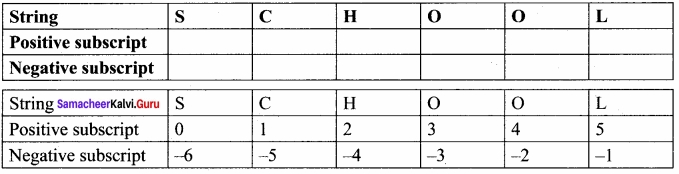Question 2.
Find the output?
Program
str1 = ‘ * ‘
i=1
while i<=5: print (str1*i)
i+=1
Output
*
* *
* * *
* * * *
* * * * *

PART – III

Question 1.
Write note on replace function?
The replace function replaces all occurrences of char 1 with char 2.
Example
>>> str1 =”How are you”
>>> print (str1)
How are you
>>>print (str1.replace(“o”, “e”))
Hew are yeuQuestion 2.
Write note on Append Operator?
Append (+ =)
Adding more strings at the end of an existing string is known as append. The operator + = is used to append a new string with an existing string.
Example:
>>> str1=’Welcome to ”
>>> str1+=”Leam Python”
>>> print (str1)
Welcome to Learn PythonQuestion 3.
Give any 6 formatting characters with their usage?
Formatting characters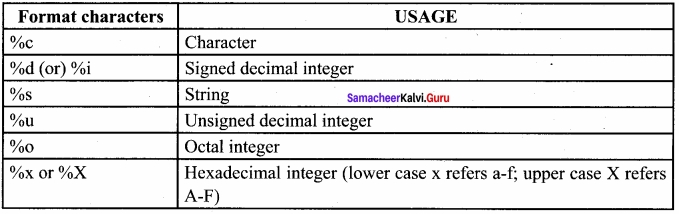Question 4.
Write any 6 escape sequences with their description?